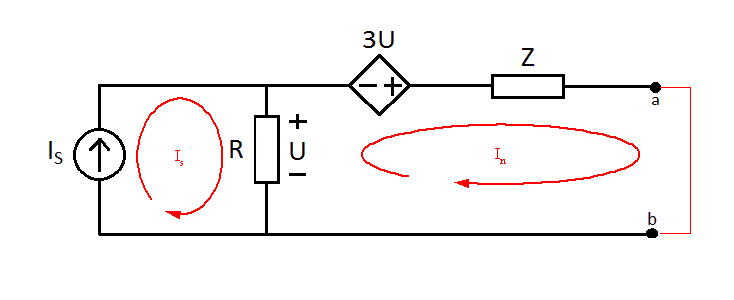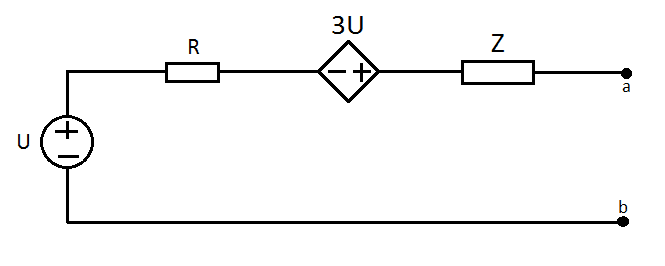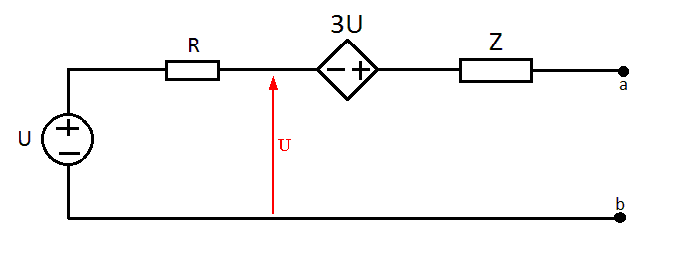# Finding the Thevenin Equivalent with a dependent source

butchsd
Hi!

I'm having some difficulty understanding how to go about solving the following problem. I'm a beginner at this and am completely lost. I've seen a few similar problems that could be solved with node/current analysis, but I don't really understand if or how to apply that here.

Homework Statement
From the attached image:
Is = 2.0∠0° A
R = 10$\Omega$
Z = j10$\Omega$

#### Attachments

•pic.png
1.7 KB · Views: 427

## Answers and Replies

Mentor
Hi!

I'm having some difficulty understanding how to go about solving the following problem. I'm a beginner at this and am completely lost. I've seen a few similar problems that could be solved with node/current analysis, but I don't really understand if or how to apply that here.

Homework Statement
From the attached image:
Is = 2.0∠0° A
R = 10$\Omega$
Z = j10$\Omega$

Hi butchsd, Welcome to Physics Forums.

You'll have to show some attempt at the problem before we can see how to help you. At the very least you can describe your understanding of Thevenin (and Norton) equivalents, the 'usual' procedure for finding them, and why this particular circuit is giving you difficulty.

One hint that you might find useful here and in future is that for a given circuit, if you divide the Thevenin voltage by the Norton current you get the Thevenin (and Norton) impedance.

butchsd
Here's what I have (or think I have) so far:

An open circuit analysis shows that the current over R is Is:
IR = 2.0∠0° A.
Voc = IR * R = 2.0∠0° * 10∠0° = 20∠0° A..

Closing the circuit from a to b and doing current division shows:
Iab = Is * R / (R+Z) = 2.0∠0° * 10 / (10+j10) = 2.0∠0° * 10∠0° / 14.14∠45° = 1.414∠-45°.

Calculating the Thevenin impedance:
Zt = Voc / Iab = 20∠0° / 1.414∠-45° = 14.14∠0°

There we have all the parts for an answer. However I have a sneaking suspicion that ignoring the dependent source like i've done is not the way to go, but I don't really understand where it should be included, or if there's a better way of going about solving this thing?

butchsd
Hmm.

Since I know that UR = 20∠0° A, I know that 3U is 60∠0° A. So then we have a Norton in serial with a Thevenin. Let's play a bit. If I first convert the circuit to two Nortons in series, maybe it'll be better.

Thevenin -> Norton for the dependent source and Z:
Isc = 60∠0° / j10 = 60∠0° / 10∠90° = 6∠-90°
Z is still Z.

Zeroing the sources would then make the total impedance:
Zt = Z+R = 10+j10.

Mesh analysis in two places gives.
UR = 20∠0° (same as before).
UZ = 6∠-90° * 10∠90° = 60∠0°

So that would make the total:
Voc = UR + UZ = 20∠0° + 60∠0° = 80∠0°

Correct or horrible? :)

Mentor
Yes, you do need to take the 3U voltage source into account. This will affect your open circuit (Thevenin) voltage since the 3U source is in series with the 'a' lead, boosting the output potential even if no current flows through the branch.

Your results so far are not correct, but you've demonstrated that you're putting in some good effort and thinking creatively about the problem. I'm not sure precisely what you had in mind by having "two Nortons in series". Perhaps you can include a diagram?

Another approach would be to insert the a-b short and do a mesh analysis; there are two loops but the first has its mesh current constrained by Is and thus is already 'solved', so you can concentrate on writing the mesh equation for the second loop; its current will be the Norton current for the circuit...With the Norton current and a (correct) Thevenin voltage in hand, you can then calculate the Thevenin impedance.

#### Attachments

butchsd
Disregard the multiple norton thing, just spitballing. :)

Let's give the mesh analysis a try. So if I solve the mesh analysis for IN I get this:

IS: IS = 2.0∠0° A

IN: -R(IS-IN) - 3U + ZIN = 0
Ohm's law:
-R(IS-IN) - 3R(IS-IN) + ZIN = 0
-4R(IS-IN) + ZIN = 0
Factor into IN and IS:
(4R+Z)IN = 4RIS
IN = 4RIS / (4R+Z)
IN = 4*10*2.0∠0° / (4*10+j10) = 80∠0° / 41.231∠14.04° = 38.769∠-14.04° A

After this I'm a bit confused. Are you saying that the 3U source affects the open circuit voltage over R even though a and b aren't shorted? If so, how? I can't find anything about this in the textbook I'm using.

Assuming the source doesn't factor in, let's continue.
Voc = R*IS = 10 * 2∠0° = 20∠0°

Zt = Voc / IN = 20∠0° / 38.769∠-14.04° = 0.52∠-14.04°

Mentor
Disregard the multiple norton thing, just spitballing. :)

Let's give the mesh analysis a try. So if I solve the mesh analysis for IN I get this:

IS: IS = 2.0∠0° A

IN: -R(IS-IN) - 3U + ZIN = 0
Ohm's law:
-R(IS-IN) - 3R(IS-IN) + ZIN = 0
-4R(IS-IN) + ZIN = 0
Factor into IN and IS:
(4R+Z)IN = 4RIS
IN = 4RIS / (4R+Z)
Fine up to this point.
IN = 4*10*2.0∠0° / (4*10+j10) = 80∠0° / 41.231∠14.04° = 38.769∠-14.04° A
Check your math in the line above. 80/41 is not close to 38...
After this I'm a bit confused. Are you saying that the 3U source affects the open circuit voltage over R even though a and b aren't shorted? If so, how? I can't find anything about this in the textbook I'm using.
Not the voltage over R, but the voltage (potential difference) between terminals a and b. The potential at a is equal to the potential at the top of R plus the potential across whatever lies between that point and terminal a. The voltage source 3U lies between...
Assuming the source doesn't factor in, let's continue.
Voc = R*IS = 10 * 2∠0° = 20∠0°

Zt = Voc / IN = 20∠0° / 38.769∠-14.04° = 0.52∠-14.04°

As explained above, you've got to take into account the 3U source when you determine Voc.

butchsd
Check your math in the line above. 80/41 is not close to 38...
Ah yes, my mistake. That should be 1.940∠-14.04° A
As explained above, you've got to take into account the 3U source when you determine Voc.

Okay so let's first determine U over R so I can determine 3U:
IR = IS - IN = 2∠0° - 1.940∠-14.04° = 2 - 1.882 - j0.471 = 0.118 - j0.471 = 0.486∠-75.9° A

U = R*IR = 10 * 0.486∠-75.9° = 4.86∠-75.9° V
3U = 14.58∠-75.9° V

Incidentally, can't I rewrite the problem like this?If so, I think I can add the sources to calculate Voc:
Voc = U + 3U = 0.118 - j0.471 + 3.552 - j14.141 = 3.670 - j14.612 = 15∠-75.9°

Assuming this is correct:
Zt = Voc / IN = 15.060∠-75.9° / 1.940∠-14.04° = 7.763∠-89.94°

Mentor
Ah yes, my mistake. That should be 1.940∠-14.04° A

Okay so let's first determine U over R so I can determine 3U:
IR = IS - IN = 2∠0° - 1.940∠-14.04° = 2 - 1.882 - j0.471 = 0.118 - j0.471 = 0.486∠-75.9° A

U = R*IR = 10 * 0.486∠-75.9° = 4.86∠-75.9° V
3U = 14.58∠-75.9° V
Ooops. For Voc, terminals a-c are open so there will be no current IN in the above.
Incidentally, can't I rewrite the problem like this?
View attachment 50096
Not quite. When you transform a circuit using an equivalent model the components are no longer the same components as the original and the "internal" parameters of the model do not necessarily correspond to those in the original circuit. Thus the 'R' in the Thevenin model is not the same as the 'R' in the original, and you can't trust the current through it or the voltage across it to be the same as in the original.

Mind you, if you keep track of the original nodes where the R was attached and use the potential between them as U, then you'll be all right.#### Attachments

butchsd
Ooops. For Voc, terminals a-c are open so there will be no current IN in the above.
Ok, so U would be R*IS = 10*2∠0° = 20∠0° V
That makes 3U = 3*20∠0° = 60∠0° V

Not quite. When you transform a circuit using an equivalent model the components are no longer the same components as the original and the "internal" parameters of the model do not necessarily correspond to those in the original circuit. Thus the 'R' in the Thevenin model is not the same as the 'R' in the original, and you can't trust the current through it or the voltage across it to be the same as in the original.

Mind you, if you keep track of the original nodes where the R was attached and use the potential between them as U, then you'll be all right.

Yeah let's just disregard the thevenin thing I tried. :)

Voc = 3U + U = 4U = 4*20∠0° = 80∠0° V = 80cos(ωt) V

Zt = Voc / IN = 80∠0° / 1.940∠-14.04° = 14.237∠14.04° Ω ≈ 40+j10 Ω

Mentor
Ok, so U would be R*IS = 10*2∠0° = 20∠0° V
That makes 3U = 3*20∠0° = 60∠0° V
Much betterYeah let's just disregard the thevenin thing I tried. :)

Voc = 3U + U = 4U = 4*20∠0° = 80∠0° V = 80cos(ωt) V

Zt = Voc / IN = 80∠0° / 1.940∠-14.04° = 14.237∠14.04° Ω ≈ 40+j10 Ω

That looks finebutchsd
Thanks for all the help :P

Mentor
Thanks for all the help :P

You're welcome. Glad to help.